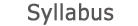2015 - 2016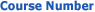0509-1824-26
Linear Algebra
FACULTY OF ENGINEERING | DEPARTMENTS OF ENGINEERING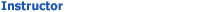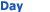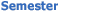Classrooms - Dan David 205 Mon 1400-1600 Sem  1
University credit hours:  2.0

Course description
Field of numbers (Rational, Real, Complex, Examples of finite fields).
Matrices, rotation matrices in the plane and in space, algebra and geometric properties. Linear equations, row operations on matrices, the row echelon form. Homogenous systems of equations, consistency conditions, general solution. Inverse matrices. Vector spaces, sub-spaces, linear dependence and linear independence. Bases and the change of bases, row spaces of matrices, column equivalence, row-column equivalence, equivalence relations and canonical forms of matrices.
Determinants: introduction as a volume function, permutations and permutation matrices, uniqueness and existence of the determinants function, practical evaluation and exterminations of transpose matrices. Cofactors, minor and adjoints, determinant and ranks. Vectors products, scalar product, the triple product and its representation as determinants, geometrical interpretation. Linear transformations and representations, rotation matrices as operators, change of bases. Eigenvalues and Eigenvectors, minors, similarity, algebra and geometric multiplicites, invariant sub-space, Jordan canonical form. Inner products, orthogonal bases, unitary equivalence and hermitian matrices. Quadric forms, positive definite matrices, orthogonal matrices as a representation of a spatial rotations, diagonolization of matrices, rotation axes, canonical forms. Classification of dyadics in the plane and in space.

accessibility declaration